# 7.1 Second-order linear equations  (Page 8/15)

 Page 8 / 15

Solve the following initial-value problem and graph the solution: $y\text{″}-2{y}^{\prime }+10y=0,y\left(0\right)=2,{y}^{\prime }\left(0\right)=-1$

$y\left(x\right)={e}^{x}\left(2\phantom{\rule{0.1em}{0ex}}\text{cos}\phantom{\rule{0.1em}{0ex}}3x-\text{sin}\phantom{\rule{0.1em}{0ex}}3x\right)$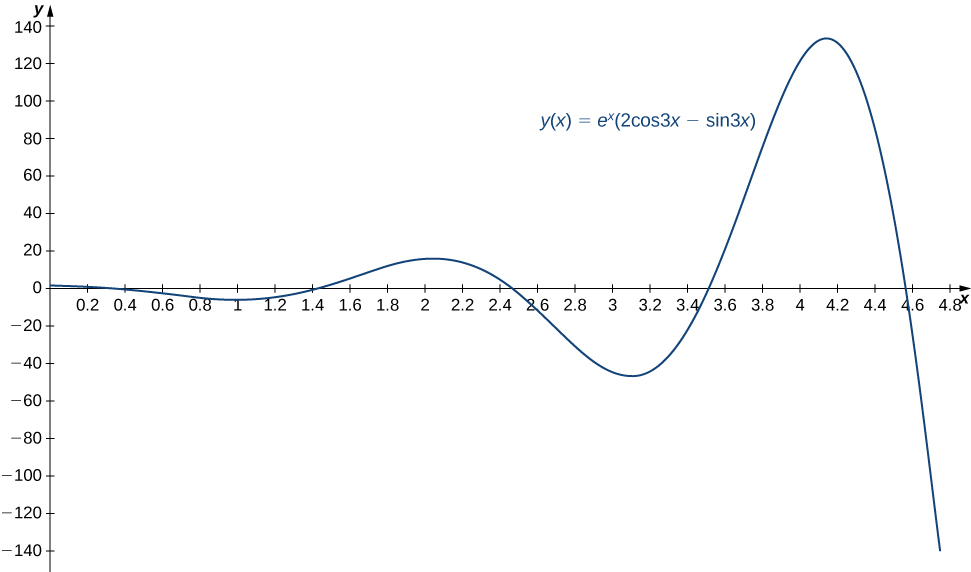## Initial-value problem representing a spring-mass system

The following initial-value problem models the position of an object with mass attached to a spring. Spring-mass systems are examined in detail in Applications . The solution to the differential equation gives the position of the mass with respect to a neutral (equilibrium) position (in meters) at any given time. (Note that for spring-mass systems of this type, it is customary to define the downward direction as positive.)

$y\text{″}+2{y}^{\prime }+y=0,y\left(0\right)=1,{y}^{\prime }\left(0\right)=0$

Solve the initial-value problem and graph the solution. What is the position of the mass at time $t=2$ sec? How fast is the mass moving at time $t=1$ sec? In what direction?

In [link] c. we found the general solution to this differential equation to be

$y\left(t\right)={c}_{1}{e}^{\text{−}t}+{c}_{2}t{e}^{\text{−}t}.$

Then

${y}^{\prime }\left(t\right)=\text{−}{c}_{1}{e}^{\text{−}t}+{c}_{2}\left(\text{−}t{e}^{\text{−}t}+{e}^{\text{−}t}\right).$

When $t=0,$ we have $y\left(0\right)={c}_{1}$ and ${y}^{\prime }\left(0\right)=\text{−}{c}_{1}+{c}_{2}.$ Applying the initial conditions, we obtain

$\begin{array}{ccc}\hfill {c}_{1}& =\hfill & 1\hfill \\ \hfill {\text{−}c}_{1}+{c}_{2}& =\hfill & 0.\hfill \end{array}$

Thus, ${c}_{1}=1,$ ${c}_{2}=1,$ and the solution to the initial value problem is

$y\left(t\right)={e}^{\text{−}t}+t{e}^{\text{−}t}.$

This solution is represented in the following graph. At time $t=2,$ the mass is at position $y\left(2\right)={e}^{-2}+2{e}^{-2}=3{e}^{-2}\approx 0.406$ m below equilibrium.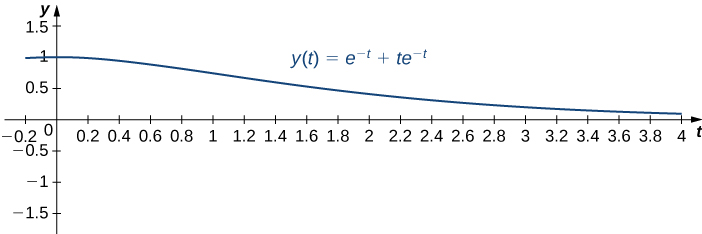To calculate the velocity at time $t=1,$ we need to find the derivative. We have $y\left(t\right)={e}^{\text{−}t}+t{e}^{\text{−}t},$ so

${y}^{\prime }\left(t\right)=\text{−}{e}^{\text{−}t}+{e}^{\text{−}t}-t{e}^{\text{−}t}=\text{−}t{e}^{\text{−}t}.$

Then ${y}^{\prime }\left(1\right)=\text{−}{e}^{-1}\approx -0.3679.$ At time $t=1,$ the mass is moving upward at 0.3679 m/sec.

Suppose the following initial-value problem models the position (in feet) of a mass in a spring-mass system at any given time. Solve the initial-value problem and graph the solution. What is the position of the mass at time $t=0.3$ sec? How fast is it moving at time $t=0.1$ sec? In what direction?

$y\text{″}+14{y}^{\prime }+49y=0,y\left(0\right)=0,{y}^{\prime }\left(0\right)=1$

$y\left(t\right)=t{e}^{-7t}$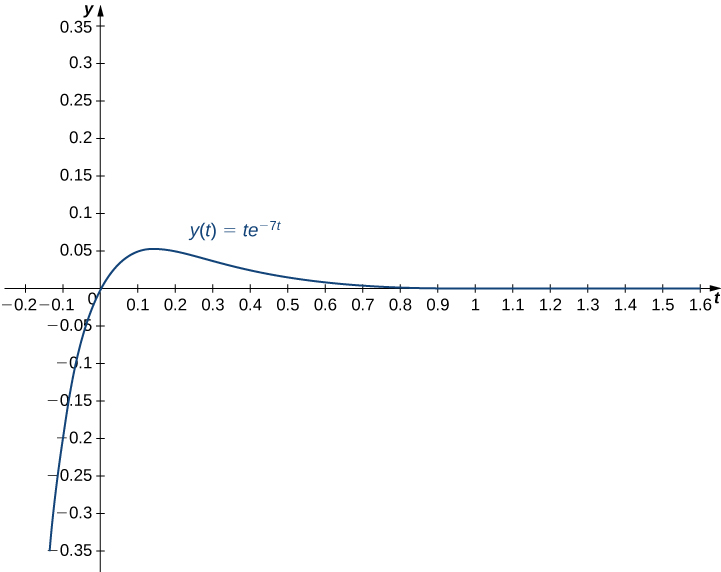At time $t=0.3,$ $y\left(0.3\right)=0.3{e}^{\left(-7*0.3\right)}=0.3{e}^{-2.1}\approx 0.0367.$ The mass is 0.0367 ft below equilibrium. At time $t=0.1,$ ${y}^{\prime }\left(0.1\right)=0.3{e}^{-0.7}\approx 0.1490.$ The mass is moving downward at a speed of 0.1490 ft/sec.

## Solving a boundary-value problem

In [link] f. we solved the differential equation $y\text{″}+16y=0$ and found the general solution to be $y\left(t\right)={c}_{1}\text{cos}\phantom{\rule{0.1em}{0ex}}4t+{c}_{2}\text{sin}\phantom{\rule{0.1em}{0ex}}4t\text{.}$ If possible, solve the boundary-value problem if the boundary conditions are the following:

1. $y\left(0\right)=0,$ $y\left(\frac{\pi }{4}\right)=0$
2. $y\left(0\right)=1,$ $y\left(\frac{\pi }{8}\right)=0$
3. $y\left(\frac{\pi }{8}\right)=0,$ $y\left(\frac{3\pi }{8}\right)=2$

We have

$y\left(x\right)={c}_{1}\text{cos}\phantom{\rule{0.1em}{0ex}}4t+{c}_{2}\text{sin}\phantom{\rule{0.1em}{0ex}}4t\text{.}$
1. Applying the first boundary condition given here, we get $y\left(0\right)={c}_{1}=0.$ So the solution is of the form $y\left(t\right)={c}_{2}\text{sin}\phantom{\rule{0.1em}{0ex}}4t\text{.}$ When we apply the second boundary condition, though, we get $y\left(\frac{\pi }{4}\right)={c}_{2}\text{sin}\phantom{\rule{0.1em}{0ex}}\left(4\left(\frac{\pi }{4}\right)\right)={c}_{2}\text{sin}\phantom{\rule{0.1em}{0ex}}\pi =0$ for all values of ${c}_{2}.$ The boundary conditions are not sufficient to determine a value for ${c}_{2},$ so this boundary-value problem has infinitely many solutions. Thus, $y\left(t\right)={c}_{2}\text{sin}\phantom{\rule{0.1em}{0ex}}4t$ is a solution for any value of ${c}_{2}.$
2. Applying the first boundary condition given here, we get $y\left(0\right)={c}_{1}=1.$ Applying the second boundary condition gives $y\left(\frac{\pi }{8}\right)={c}_{2}=0,$ so ${c}_{2}=0.$ In this case, we have a unique solution: $y\left(t\right)=\text{cos}\phantom{\rule{0.1em}{0ex}}4t\text{.}$
3. Applying the first boundary condition given here, we get $y\left(\frac{\pi }{8}\right)={c}_{2}=0.$ However, applying the second boundary condition gives $y\left(\frac{3\pi }{8}\right)=\text{−}{c}_{2}=2,$ so ${c}_{2}=-2.$ We cannot have ${c}_{2}=0=-2,$ so this boundary value problem has no solution.

## Key concepts

• Second-order differential equations can be classified as linear or nonlinear, homogeneous or nonhomogeneous.
• To find a general solution for a homogeneous second-order differential equation, we must find two linearly independent solutions. If ${y}_{1}\left(x\right)$ and ${y}_{2}\left(x\right)$ are linearly independent solutions to a second-order, linear, homogeneous differential equation, then the general solution is given by
$y\left(x\right)={c}_{1}{y}_{1}\left(x\right)+{c}_{2}{y}_{2}\left(x\right).$
• To solve homogeneous second-order differential equations with constant coefficients, find the roots of the characteristic equation. The form of the general solution varies depending on whether the characteristic equation has distinct, real roots; a single, repeated real root; or complex conjugate roots.
• Initial conditions or boundary conditions can then be used to find the specific solution to a differential equation that satisfies those conditions, except when there is no solution or infinitely many solutions.

## Key equations

• Linear second-order differential equation
${a}_{2}\left(x\right)y\text{″}+{a}_{1}\left(x\right){y}^{\prime }+{a}_{0}\left(x\right)y=r\left(x\right)$
• Second-order equation with constant coefficients
$ay\text{″}+b{y}^{\prime }+cy=0$

Classify each of the following equations as linear or nonlinear. If the equation is linear, determine whether it is homogeneous or nonhomogeneous.

${x}^{3}y\text{″}+\left(x-1\right){y}^{\prime }-8y=0$

linear, homogenous

$\left(1+{y}^{2}\right)y\text{″}+x{y}^{\prime }-3y=\text{cos}\phantom{\rule{0.1em}{0ex}}x$

$xy\text{″}+{e}^{y}{y}^{\prime }=x$

nonlinear

$y\text{″}+\frac{4}{x}{y}^{\prime }-8xy=5{x}^{2}+1$

$y\text{″}+\left(\text{sin}\phantom{\rule{0.1em}{0ex}}x\right){y}^{\prime }-xy=4y$

linear, homogeneous

$y\text{″}+\left(\frac{x+3}{y}\right){y}^{\prime }=0$

For each of the following problems, verify that the given function is a solution to the differential equation. Use a graphing utility to graph the particular solutions for several values of c 1 and c 2 . What do the solutions have in common?

[T] $y\text{″}+2{y}^{\prime }-3y=0;$ $y\left(x\right)={c}_{1}{e}^{x}+{c}_{2}{e}^{-3x}$

[T] ${x}^{2}y\text{″}-2y-3{x}^{2}+1=0;$ $y\left(x\right)={c}_{1}{x}^{2}+{c}_{2}{x}^{-1}+{x}^{2}\text{ln}\phantom{\rule{0.1em}{0ex}}\left(x\right)+\frac{1}{2}$

[T] $y\text{″}+14{y}^{\prime }+49y=0;$ $y\left(x\right)={c}_{1}{e}^{-7x}+{c}_{2}x{e}^{-7x}$

[T] $6y\text{″}-49{y}^{\prime }+8y=0;$ $y\left(x\right)={c}_{1}{e}^{x\text{/}6}+{c}_{2}{e}^{8x}$

Find the general solution to the linear differential equation.

$y\text{″}-3{y}^{\prime }-10y=0$

$y={c}_{1}{e}^{5x}+{c}_{2}{e}^{-2x}$

$y\text{″}-7{y}^{\prime }+12y=0$

$y\text{″}+4{y}^{\prime }+4y=0$

$y={c}_{1}{e}^{-2x}+{c}_{2}x{e}^{-2x}$

$4y\text{″}-12{y}^{\prime }+9y=0$

$2y\text{″}-3{y}^{\prime }-5y=0$

$y={c}_{1}{e}^{5x\text{/}2}+{c}_{2}{e}^{\text{−}x}$

$3y\text{″}-14{y}^{\prime }+8y=0$

$y\text{″}+{y}^{\prime }+y=0$

$y={e}^{\text{−}x\text{/}2}\left({c}_{1}\text{cos}\phantom{\rule{0.1em}{0ex}}\frac{\sqrt{3}x}{2}+{c}_{2}\text{sin}\phantom{\rule{0.1em}{0ex}}\frac{\sqrt{3}x}{2}\right)$

$5y\text{″}+2{y}^{\prime }+4y=0$

$y\text{″}-121y=0$

$y={c}_{1}{e}^{-11x}+{c}_{2}{e}^{11x}$

$8y\text{″}+14{y}^{\prime }-15y=0$

$y\text{″}+81y=0$

$y={c}_{1}\text{cos}\phantom{\rule{0.1em}{0ex}}9x+{c}_{2}\text{sin}\phantom{\rule{0.1em}{0ex}}9x$

$y\text{″}-{y}^{\prime }+11y=0$

$2y\text{″}=0$

$y={c}_{1}+{c}_{2}x$

$y\text{″}-6{y}^{\prime }+9y=0$

$3y\text{″}-2{y}^{\prime }-7y=0$

$y={c}_{1}{e}^{\left(\left(1+\sqrt{22}\right)\text{/}3\right)x}+{c}_{2}{e}^{\left(\left(1-\sqrt{22}\right)\text{/}3\right)x}$

$4y\text{″}-10{y}^{\prime }=0$

$36\frac{{d}^{2}y}{d{x}^{2}}+12\frac{dy}{dx}+y=0$

$y={c}_{1}{e}^{\text{−}x\text{/}6}+{c}_{2}x{e}^{\text{−}x\text{/}6}$

$25\frac{{d}^{2}y}{d{x}^{2}}-80\frac{dy}{dx}+64y=0$

$\frac{{d}^{2}y}{d{x}^{2}}-9\frac{dy}{dx}=0$

$y={c}_{1}+{c}_{2}{e}^{9x}$

$4\frac{{d}^{2}y}{d{x}^{2}}+8y=0$

Solve the initial-value problem.

$y\text{″}+5{y}^{\prime }+6y=0,\phantom{\rule{2em}{0ex}}y\left(0\right)=0,\phantom{\rule{0.2em}{0ex}}\text{}\phantom{\rule{0.2em}{0ex}}{y}^{\prime }\left(0\right)=-2$

$y=-2{e}^{-2x}+2{e}^{-3x}$

$y\text{″}+2{y}^{\prime }-8y=0,\phantom{\rule{2em}{0ex}}y\left(0\right)=5,\phantom{\rule{0.2em}{0ex}}\text{}\phantom{\rule{0.2em}{0ex}}{y}^{\prime }\left(0\right)=4$

$y\text{″}+4y=0,\phantom{\rule{4em}{0ex}}y\left(0\right)=3,\phantom{\rule{0.2em}{0ex}}\text{}\phantom{\rule{0.2em}{0ex}}{y}^{\prime }\left(0\right)=10$

$y=3\phantom{\rule{0.1em}{0ex}}\text{cos}\phantom{\rule{0.1em}{0ex}}\left(2x\right)+5\phantom{\rule{0.1em}{0ex}}\text{sin}\phantom{\rule{0.1em}{0ex}}\left(2x\right)$

$y\text{″}-18{y}^{\prime }+81y=0,\phantom{\rule{2em}{0ex}}y\left(0\right)=1,\phantom{\rule{0.2em}{0ex}}\text{}\phantom{\rule{0.2em}{0ex}}{y}^{\prime }\left(0\right)=5$

$y\text{″}-{y}^{\prime }-30y=0,\phantom{\rule{2em}{0ex}}y\left(0\right)=1,\phantom{\rule{0.2em}{0ex}}\text{}\phantom{\rule{0.2em}{0ex}}{y}^{\prime }\left(0\right)=-16$

$y=\text{−}{e}^{6x}+2{e}^{-5x}$

$4y\text{″}+4{y}^{\prime }-8y=0,\phantom{\rule{2em}{0ex}}y\left(0\right)=2,\phantom{\rule{0.2em}{0ex}}\text{}\phantom{\rule{0.2em}{0ex}}{y}^{\prime }\left(0\right)=1$

$25y\text{″}+10{y}^{\prime }+y=0,\phantom{\rule{2em}{0ex}}y\left(0\right)=2,\phantom{\rule{0.2em}{0ex}}\text{}\phantom{\rule{0.2em}{0ex}}{y}^{\prime }\left(0\right)=1$

$y=2{e}^{\text{−}x\text{/}5}+\frac{7}{5}x{e}^{\text{−}x\text{/}5}$

$y\text{″}+y=0,\phantom{\rule{4em}{0ex}}y\left(\pi \right)=1,\phantom{\rule{0.2em}{0ex}}\text{}\phantom{\rule{0.2em}{0ex}}{y}^{\prime }\left(\pi \right)=-5$

Solve the boundary-value problem, if possible.

$y\text{″}+{y}^{\prime }-42y=0,\phantom{\rule{2em}{0ex}}y\left(0\right)=0,\phantom{\rule{0.2em}{0ex}}\text{}\phantom{\rule{0.2em}{0ex}}y\left(1\right)=2$

$y=\left(\frac{2}{{e}^{6}-{e}^{-7}}\right){e}^{6x}-\left(\frac{2}{{e}^{6}-{e}^{-7}}\right){e}^{-7x}$

$9y\text{″}+y=0,\phantom{\rule{4em}{0ex}}y\left(\frac{3\pi }{2}\right)=6,\phantom{\rule{0.2em}{0ex}}\text{}\phantom{\rule{0.2em}{0ex}}y\left(0\right)=-8$

$y\text{″}+10{y}^{\prime }+34y=0,\phantom{\rule{2em}{0ex}}y\left(0\right)=6,\phantom{\rule{0.2em}{0ex}}\text{}\phantom{\rule{0.2em}{0ex}}y\left(\pi \right)=2$

No solutions exist.

$y\text{″}+7{y}^{\prime }-60y=0,\phantom{\rule{2em}{0ex}}y\left(0\right)=4,\phantom{\rule{0.2em}{0ex}}\text{}\phantom{\rule{0.2em}{0ex}}y\left(2\right)=0$

$y\text{″}-4{y}^{\prime }+4y=0,\phantom{\rule{2em}{0ex}}y\left(0\right)=2,\phantom{\rule{0.2em}{0ex}}\text{}\phantom{\rule{0.2em}{0ex}}y\left(1\right)=-1$

$y=2{e}^{2x}-\frac{2{e}^{2}+1}{{e}^{2}}x{e}^{2x}$

$y\text{″}-5{y}^{\prime }=0,\phantom{\rule{2em}{0ex}}y\left(0\right)=3,\phantom{\rule{0.2em}{0ex}}\text{}\phantom{\rule{0.2em}{0ex}}y\left(-1\right)=2$

$y\text{″}+9y=0,\phantom{\rule{2em}{0ex}}y\left(0\right)=4,\phantom{\rule{0.2em}{0ex}}\text{}\phantom{\rule{0.2em}{0ex}}y\left(\frac{\pi }{3}\right)=-4$

$y=4\phantom{\rule{0.1em}{0ex}}\text{cos}\phantom{\rule{0.1em}{0ex}}3x+{c}_{2}\text{sin}\phantom{\rule{0.1em}{0ex}}3x,\phantom{\rule{0.2em}{0ex}}\text{infinitely many solutions}$

$4y\text{″}+25y=0,\phantom{\rule{2em}{0ex}}y\left(0\right)=2,\phantom{\rule{0.2em}{0ex}}\text{}\phantom{\rule{0.2em}{0ex}}y\left(2\pi \right)=-2$

Find a differential equation with a general solution that is $y={c}_{1}{e}^{x\text{/}5}+{c}_{2}{e}^{-4x}.$

$5y\text{″}+19{y}^{\prime }-4y=0$

Find a differential equation with a general solution that is $y={c}_{1}{e}^{x}+{c}_{2}{e}^{-4x\text{/}3}.$

For each of the following differential equations:

1. Solve the initial value problem.
2. [T] Use a graphing utility to graph the particular solution.

$y\text{″}+64y=0;\phantom{\rule{2em}{0ex}}y\left(0\right)=3,\phantom{\rule{1em}{0ex}}{y}^{\prime }\left(0\right)=16$

a. $y=3\phantom{\rule{0.1em}{0ex}}\text{cos}\left(8x\right)+2\phantom{\rule{0.1em}{0ex}}\text{sin}\left(8x\right)$
b.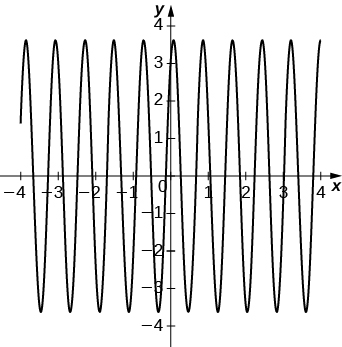$y\text{″}-2{y}^{\prime }+10y=0\phantom{\rule{2em}{0ex}}y\left(0\right)=1,\phantom{\rule{1em}{0ex}}{y}^{\prime }\left(0\right)=13$

$y\text{″}+5{y}^{\prime }+15y=0\phantom{\rule{2em}{0ex}}y\left(0\right)=-2,\phantom{\rule{1em}{0ex}}{y}^{\prime }\left(0\right)=7$

a. $y={e}^{\left(-5\text{/}2\right)x}\left[-2\phantom{\rule{0.1em}{0ex}}\text{cos}\phantom{\rule{0.1em}{0ex}}\left(\frac{\sqrt{35}}{2}x\right)+\frac{4\sqrt{35}}{35}\phantom{\rule{0.1em}{0ex}}\text{sin}\phantom{\rule{0.1em}{0ex}}\left(\frac{\sqrt{35}}{2}x\right)\right]$
b.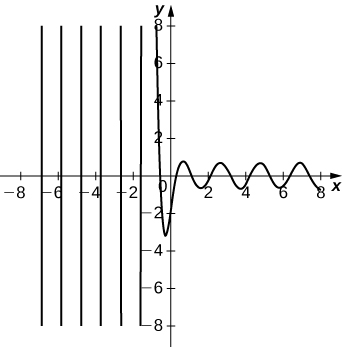(Principle of superposition) Prove that if ${y}_{1}\left(x\right)$ and ${y}_{2}\left(x\right)$ are solutions to a linear homogeneous differential equation, $y\text{″}+p\left(x\right){y}^{\prime }+q\left(x\right)y=0,$ then the function $y\left(x\right)={c}_{1}{y}_{1}\left(x\right)+{c}_{2}{y}_{2}\left(x\right),$ where ${c}_{1}$ and ${c}_{2}$ are constants, is also a solution.

Prove that if a, b, and c are positive constants, then all solutions to the second-order linear differential equation $ay\text{″}+b{y}^{\prime }+cy=0$ approach zero as $x\to \infty \text{.}$ ( Hint: Consider three cases: two distinct roots, repeated real roots, and complex conjugate roots.)

are nano particles real
yeah
Joseph
Hello, if I study Physics teacher in bachelor, can I study Nanotechnology in master?
no can't
Lohitha
where we get a research paper on Nano chemistry....?
nanopartical of organic/inorganic / physical chemistry , pdf / thesis / review
Ali
what are the products of Nano chemistry?
There are lots of products of nano chemistry... Like nano coatings.....carbon fiber.. And lots of others..
learn
Even nanotechnology is pretty much all about chemistry... Its the chemistry on quantum or atomic level
learn
da
no nanotechnology is also a part of physics and maths it requires angle formulas and some pressure regarding concepts
Bhagvanji
hey
Giriraj
Preparation and Applications of Nanomaterial for Drug Delivery
revolt
da
Application of nanotechnology in medicine
has a lot of application modern world
Kamaluddeen
yes
narayan
what is variations in raman spectra for nanomaterials
ya I also want to know the raman spectra
Bhagvanji
I only see partial conversation and what's the question here!
what about nanotechnology for water purification
please someone correct me if I'm wrong but I think one can use nanoparticles, specially silver nanoparticles for water treatment.
Damian
yes that's correct
Professor
I think
Professor
Nasa has use it in the 60's, copper as water purification in the moon travel.
Alexandre
nanocopper obvius
Alexandre
what is the stm
is there industrial application of fullrenes. What is the method to prepare fullrene on large scale.?
Rafiq
industrial application...? mmm I think on the medical side as drug carrier, but you should go deeper on your research, I may be wrong
Damian
How we are making nano material?
what is a peer
What is meant by 'nano scale'?
What is STMs full form?
LITNING
scanning tunneling microscope
Sahil
how nano science is used for hydrophobicity
Santosh
Do u think that Graphene and Fullrene fiber can be used to make Air Plane body structure the lightest and strongest. Rafiq
Rafiq
what is differents between GO and RGO?
Mahi
what is simplest way to understand the applications of nano robots used to detect the cancer affected cell of human body.? How this robot is carried to required site of body cell.? what will be the carrier material and how can be detected that correct delivery of drug is done Rafiq
Rafiq
if virus is killing to make ARTIFICIAL DNA OF GRAPHENE FOR KILLED THE VIRUS .THIS IS OUR ASSUMPTION
Anam
analytical skills graphene is prepared to kill any type viruses .
Anam
Any one who tell me about Preparation and application of Nanomaterial for drug Delivery
Hafiz
what is Nano technology ?
write examples of Nano molecule?
Bob
The nanotechnology is as new science, to scale nanometric
brayan
nanotechnology is the study, desing, synthesis, manipulation and application of materials and functional systems through control of matter at nanoscale
Damian
Is there any normative that regulates the use of silver nanoparticles?
what king of growth are you checking .?
Renato
can you provide the details of the parametric equations for the lines that defince doubly-ruled surfeces (huperbolids of one sheet and hyperbolic paraboloid). Can you explain each of the variables in the equations?

#### Get Jobilize Job Search Mobile App in your pocket Now!By Brooke DelaneyByBy OpenStaxBy Dionne MahaffeyBy Madison ChristianBy OpenStaxBy Christine ZeelieBy Madison ChristianBy Hoy WenBy Robert Morris